# Mathematics - Signals And Systems - Exercises on Sampling and Interpolation[Image1]

## Introduction

Hey it's a me again @drifter1!

Today we continue with my mathematics series about Signals and Systems in order to cover Exercises on Sampling and Interpolation.

So, without further ado, let's dive straight into it!

## Sampling Example [Based on 16.2 from Ref1]

Consider the system shown below: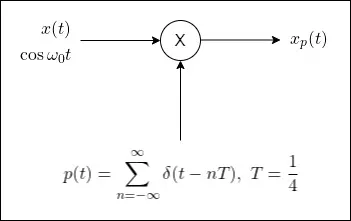Sketch the Fourier Transform of the output,, in the range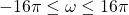for various values of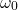, in order to identify at least one value pair with identical(or).

### Solution

The Fourier Transform of the sampling function
, which is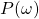, is given by:Graphically, there are basically impulses of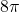every, as shown below: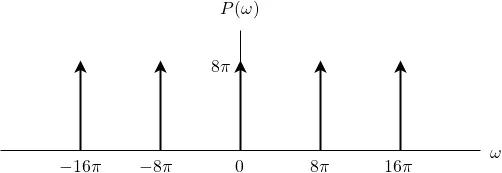Now for the input signal,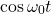. Its spectrum is a sum of two impulses,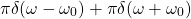, and so the plot is as follows: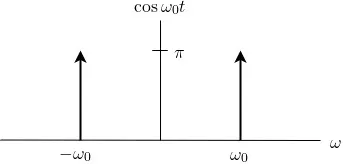Based on the convolution theorem, its straighforward to plot, because its simply:
This basically means that the graph ofis repeated at the center of each impulse of, giving one inpulse to the left, and one impulse to the right, with a distance that depends on the value of.

As such, in the case of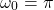, the output is:For: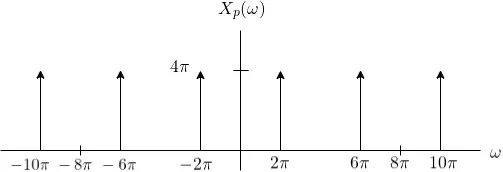For: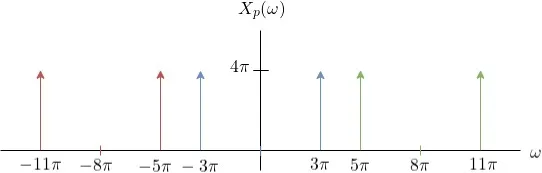For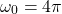adjacent impulse pairs "fuse" together leading to double the magnitude: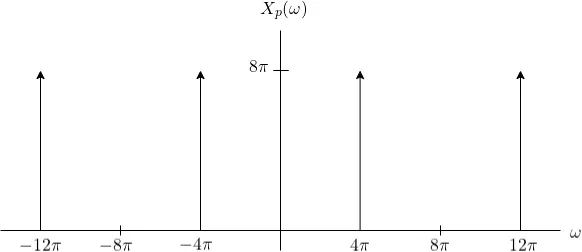Lastly, forwe get the same output as with, because the impulse pairs "overlap" each other, as shown below with easy-to-see colour coding: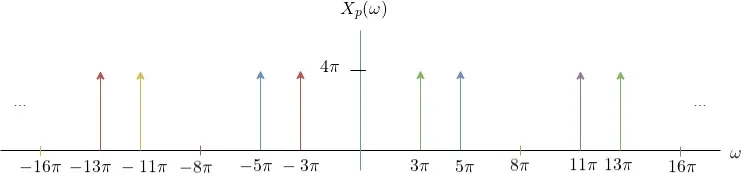The same also occurs in the case of the value pair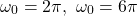, and also for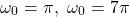.

## Interpolation Example [Based on 17.2 from Ref1]

Consider the signal, which we want to interpolate using the following generic LTI system:Let's sketch out the output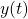for the following choices of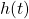: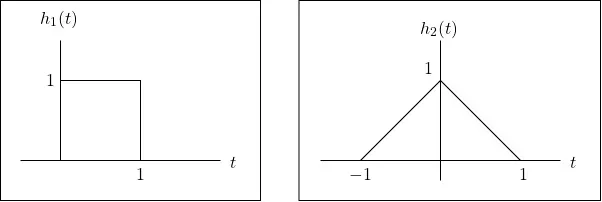### Solution

The signal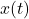can be visualized as follows: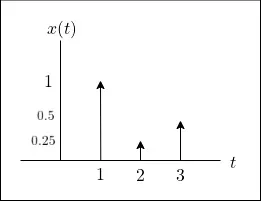For both choices of, the output is simply the superposition of the two signals. Therefore, the output can be sketched quite easily, as seen below: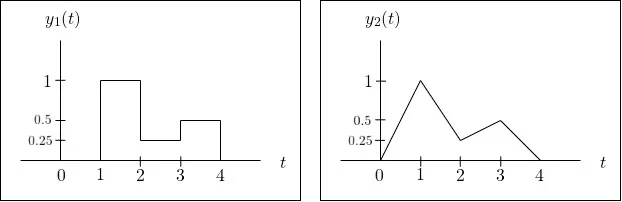## RESOURCES:

### Images

1.Block diagrams and other visualizations were made using draw.io and GeoGebra

## Final words | Next up

And this is actually it for today's post!

From next time on we will start getting into other kinds of Transforms, which will also lead to the end of this amazing series...

See Ya!Keep on drifting!

H2
H3
H4
3 columns
2 columns
1 column
1 Comment